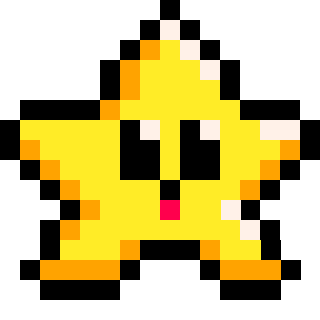# CUSTOM_FUNCTIONS

``function name_of_function()``

You may want to create your own functions to help organize your code and make your code more efficient.

Here's an example of a simple custom function that adds two numbers together:

``````function addNumbers(num1, num2)
sum = num1 + num2
return sum
end``````

In this function, we're using the `function` keyword to define a new function called `addNumbers`. The function takes two parameters: `num1` and `num2`, which represent the two numbers we want to add together. Inside the function, we're adding the two numbers together and storing the result in a new variable called sum. Finally, we're using the `return` keyword to send the sum variable back to wherever we asked the function to run.

To run this function, we could write some code like this:

``````result = addNumbers(5, 10)
print(result)
--prints 15``````

This code can be written anywhere else inside our game code. We are creating a variable named `result`and at the same time we're calling the `addNumbers` function to run and we pass the numbers `5` and `10` to the function inside of the parentheses. The function then accepts those numbers in the exact order they were given, so that `num1` becomes `5`, and `num2` becomes `10`. This function will then create a variable named `sum`, add `num1` and `num2` together, then return that sum as the result. So it will add `5` with `10`, and save `15` to `sum`. Then it returns `sum` to where we called it.

The returned value from the `addnumbers` function is then saved as `result`. Finally, we're using the print function to display the value of result on the screen.533

4 Nov 2023

`Font`